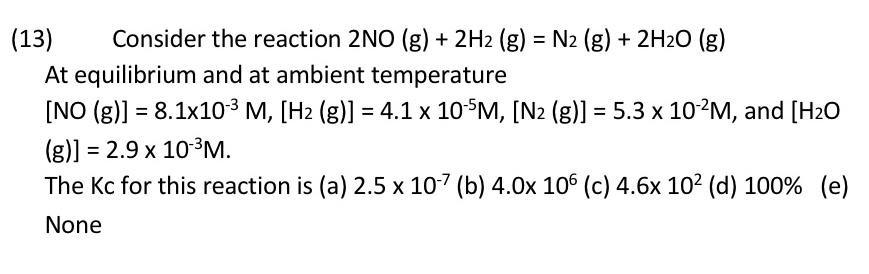Question:

# = = (13) Consider the reaction 2NO(g) + 2H2 (g) = N2 (g) + 2H20 (g) At equilibrium and at ambient temperature [NO (g)] = 8.1x10-= = (13) Consider the reaction 2NO(g) + 2H2 (g) = N2 (g) + 2H20 (g) At equilibrium and at ambient temperature [NO (g)] = 8.1x10-3 M, [H2 (g)] = 4.1 x 10-5M, [N2 (g)] = 5.3 x 10-2M, and [H20 (g)] = 2.9 x 10-3M. x The Kc for this reaction is (a) 2.5 x 10-7(b) 4.0x 106 (c) 4.6x 102 (d) 100% (e) None =